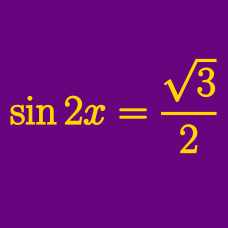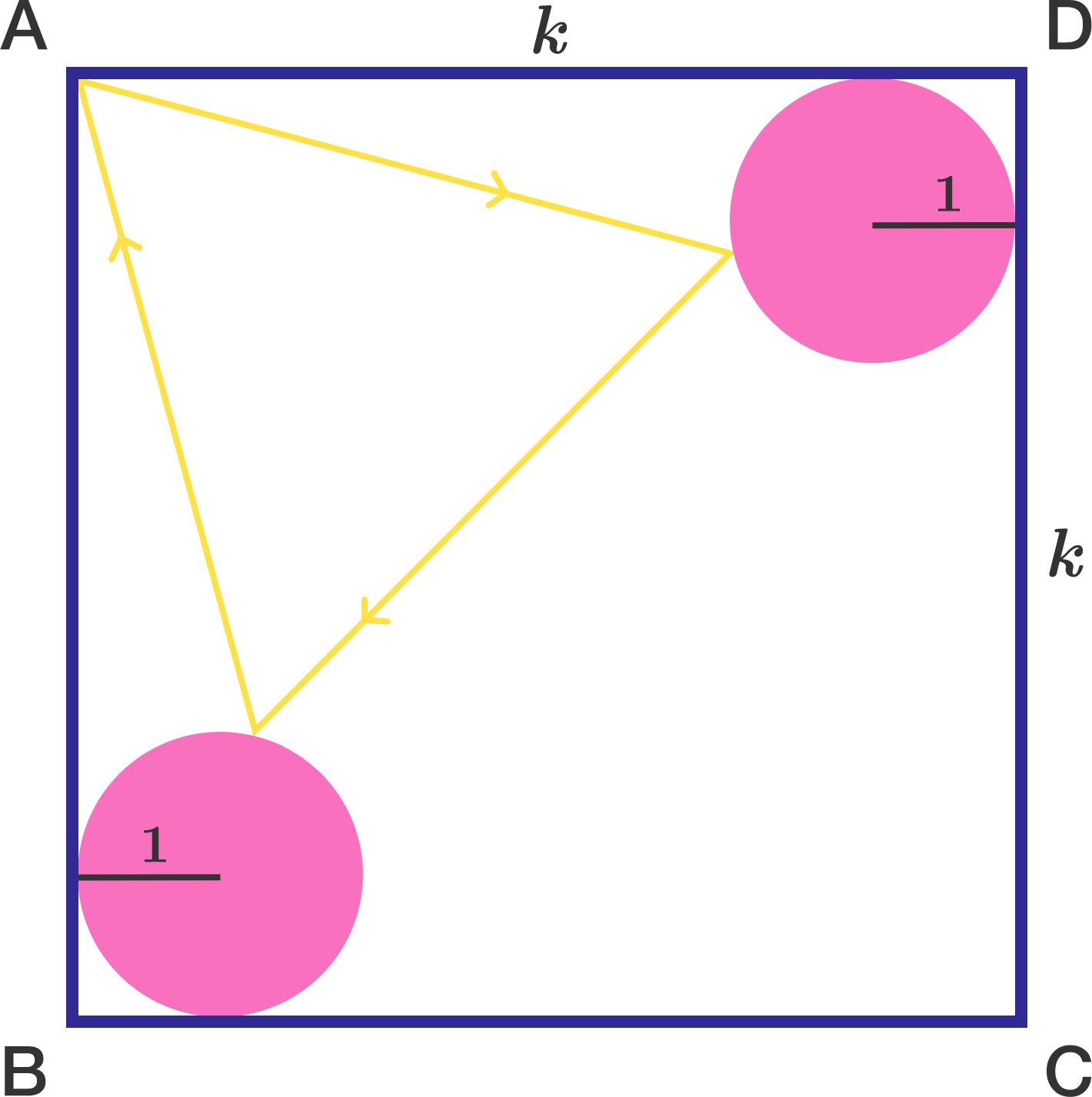Geometry

# Trigonometric Equations: Level 4 Challenges

Find the smallest possible positive value of $$\theta$$ such that $\tan^2(\theta)=\sec (\theta).$

If $$\theta$$ can be represented by $$\tan^{-1}\left( \ \sqrt{\dfrac{a+\sqrt{b}}{c}}\ \right)$$ where $$b$$ is square free and $$a,b,c$$ are integers. Find $$a+b+c$$.

How many real numbers $$x$$ satisfy

$\pi \cdot \sin x= 2x?$

A square $$ABCD$$ of side length $$k$$ contains unit circles at each of corners $$B$$ and $$D$$ such that each circle is tangent to the square at precisely two points. A ray of light emanating from point $$A$$ reflects off each circle and then returns to $$A$$, creating a path in the shape of an equilateral triangle.

There is a unique value of $$k$$ for which this scenario can occur. Find $$\lfloor 10000\cdot k \rfloor$$.

Note: "Reflecting" means that the angle of incidence equals the angle of reflection.It is the case that $$S = \displaystyle\sum_{n=-1}^{2015} \csc((2^{n+4})^{\circ}) = \sec(k^{\circ})$$ for some integer $$0 \lt k \lt 90$$. Find $$k$$.

Find the sum of all $$\theta$$ such that $\sin ^8 {\theta} + \cos ^8 {\theta} = \frac{17}{32} \quad \text{where } 0 \leq \theta \leq \pi.$

×

Problem Loading...

Note Loading...

Set Loading...